You are here: Home / Support / HOWTOs /
2023-12-10 - 08:25

## Dates and Events:

2023-11-12 12:00

### Open Source License Obligations Checklists even better now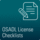Import the checklists to other tools, create context diffs and merged lists

2023-03-01 12:00

### Embedded Linux distributions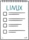Results of the online "wish list"

2022-07-11 12:00

### Call for participation in phase #4 of Open Source OPC UA open62541 support project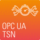Letter of Intent fulfills wish list from recent survey

2022-01-13 12:00

### Phase #3 of OSADL project on OPC UA PubSub over TSN successfully completed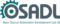Another important milestone on the way to interoperable Open Source real-time Ethernet has been reached

2021-02-09 12:00

### Open Source OPC UA PubSub over TSN project phase #3 launchedLetter of Intent with call for participation is now available

2017-09-12 12:00Facilitate Open Source software delivery

# Create a set of test data

In a first step, we need to create some test data that must be formatted in the same way as the actual plot data:

`<Number (µs)> <space> <frequency core #0> <tabulator> <frequency core #1> <tabulator> <frequency core #N>`

To do so, we run the following script:

`#!/bin/bashecho=`which echo`for i in `seq 1 180`do  core0=`expr 90000000 - \$i \* 360000`  core1=`expr 80000000 - \$i \* 360000`  core2=`expr 70000000 - \$i \* 360000`  core3=`expr 80000000 - \$i \* 360000`  rm -f latency-\$i  for j in `seq 0 399`  do    \$echo -e `printf %06d \$j` \$core0\\t\$core1\\t\$core2\\t\$core3 >>latency-`printf %03d \$i`    core0=`expr \$core0 - 500000`; if test \$core0 -lt 0; then core0=0; fi;    core1=`expr \$core1 - 500000`; if test \$core1 -lt 0; then core1=0; fi;    core2=`expr \$core2 - 500000`; if test \$core2 -lt 0; then core2=0; fi;    core3=`expr \$core3 - 500000`; if test \$core3 -lt 0; then core3=0; fi;  donedonesed -i "s/200 0/200 1/" latency-090`

which will create a total of 180 simulated data files named latency-001 to latency-180 in the same format as if they had been obtained form cyclictest running in histogram mode on a 4-core processor. An artificial single outlier with a latency of 200 µs occurring at latency plot #90 is inserted.

# Create the pseudo 3D graphic with R

This R script will read the data, select the highest value per line, transform them to a 3D matrix and call the persp() routine to generate the graphic.

`read.matrix <-function(file, header = FALSE, sep = "", skip = 0){  row.lens <- count.fields(file, sep = sep, skip = skip)  if(any(row.lens != row.lens))     stop("number of columns is not constant")  if(header) {    nrows <- length(row.lens) - 1    ncols <- row.lens    col.names <- scan(file, what = "", sep = sep, nlines = 1, quiet = TRUE, skip = skip)    x <- scan(file, sep = sep, skip = skip + 1, quiet = TRUE)  }  else {    nrows <- length(row.lens)    ncols <- row.lens    x <- scan(file, sep = sep, skip = skip, quiet = TRUE)    col.names <- NULL  }  x <- as.double(x)  if(ncols > 1) {    dim(x) <- c(ncols,nrows)    x <- t(x)    colnames(x) <- col.names  }  else if(ncols == 1)    x <- as.vector(x)  else    stop("wrong number of columns")  return(x)}read.latencyplots <-function(dir = "."){  i <- 1  files <- dir(".")  files <- sort(files, decreasing = TRUE)  r = read.delim(files, sep = "\n")  rows = dim(r) + 1  z <- matrix(nrow = rows, ncol = length(files))  for (file in files) {    m <- read.matrix(file=file)    m[,1] <- 0    m <- apply(m, 1, max, na.rm = TRUE)    z[,i] <- m    i <- i + 1  }  return(z)}data = read.latencyplots(dir = ".")data <- data + 0.1data <- log10(data)z <- datax <- 1:nrow(z)x <- c(min(x) - 1e-10, x, max(x) + 1e-10)y <- 1:ncol(z)y <- c(min(y) - 1e-10, y, max(y) + 1e-10)z0 <- min(z)z <- rbind(z0, cbind(z0, z, z0), z0)fill <- matrix("green3", nrow = nrow(z)-1, ncol = ncol(z)-1)fill[ , i2 <- c(1,ncol(fill))] <- "gray"fill[i1 <- c(1,nrow(fill)) , ] <- "gray"par(bg = "slategray")fillfunc <- colorRampPalette(c("green", "yellow", "red"), space = "Lab",  interpolate = "spline")fcol <- fill ; fcol[] <- fillfunc(nrow(fcol))pdf("../3Dplot.pdf", paper = "special", width = 11.69, height = 8.26)par(fin = c(10.69, 7.26), mai = c(0.5, 0.5, 0.5, 0.5), ps = 12, pty = "m")persp(x, y, z, theta = 30, phi = 25, col = fcol, scale = FALSE, expand = 10 * nrow(z) / 200, nticks = 10, ticktype = "detailed",  ltheta = -70, shade = 0.4, border = NA, box = TRUE, axes = TRUE, xlab = "Latency (us)", r = sqrt(3),  zlab = "Frequency (log10)", ylab = "Repetitions")title(main = "3D plot", font.main = 4)`

# Run the scripts and display the result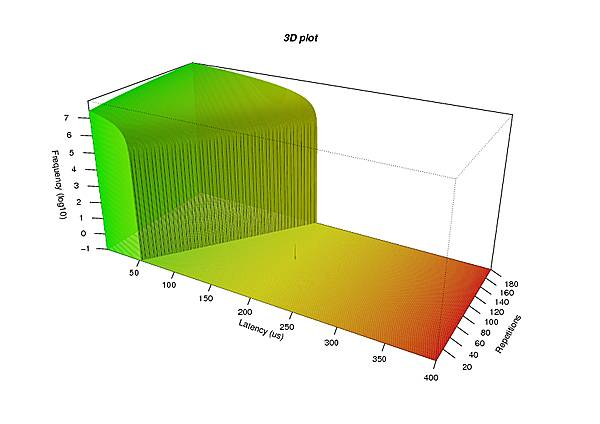Output of the R script (click on the image to show the PDF file)

`cd /tmpwget https://www.osadl.org/uploads/media/mkplotdata.bashchmod +x mkplotdata.bashwget https://www.osadl.org/uploads/media/mk3dplot.Rmkdir 3Dcd 3D../mkplotdata.bashR -f ../mk3dplot.Rcd ..evince 3Dplot.pdf`

and a graphic similar to the one at the right will be displayed. It may then further be processed with usual image converters to create bitmap files in a desired format.

Note that – due to the logarithmic vertical scale – the single outlier in the amount of 200 µs is distinguishable out of the many million recordings at latency plot #90.